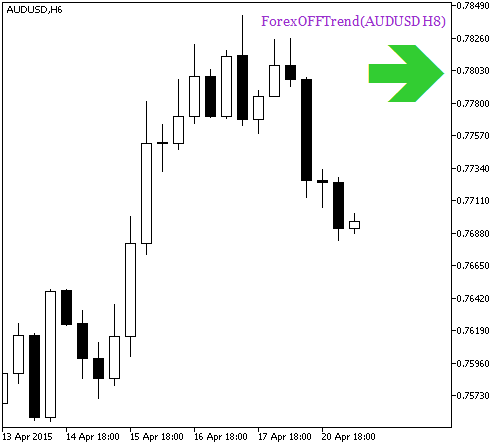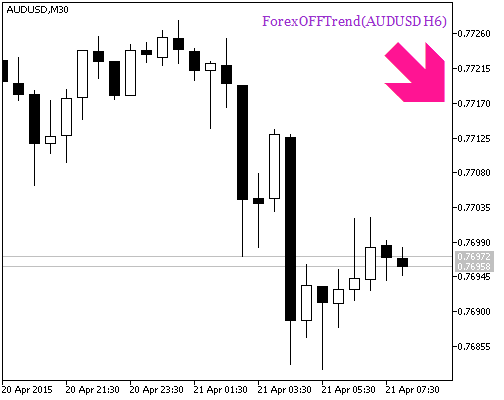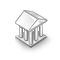584

2015.06.04 14:14

2016.11.22 07:33

1. ForexOFFTrendSign 的输入参数:
```input string Symbol_="";                               // 金融资产
input ENUM_TIMEFRAMES Timeframe=PERIOD_H6;             // 指标计算时间帧
input uint    SSP = 7;                                 // 搜索极值点的周期
input uint   KPer = 7;                                 // 信号包络移位周期
input double Kmax = 50.6;
```
2. ForexOFFTrend_HTF_Signal 指标所需要的可视化输入参数:
```//---- 指标显示设置
input uint SignalBar=0;                                // 信号得到的柱线数量 (0 - 当前柱线)
input string Symbols_Sirname=INDICATOR_NAME"_Label_";  // 指标标签名
input color Upsymbol_Color=clrLimeGreen;               // 上行趋势符号颜色
input color Dnsymbol_Color=clrDeepPink;                // 下行趋势符号颜色
input color IndName_Color=clrDarkOrchid;               // 指标名称颜色
input uint Symbols_Size=60;                            // 信号符号大小
input uint Font_Size=10;                               // 指标名称字体大小
input int X_1=5;                                       // 水平名称偏移
input int Y_1=-15;                                     // 垂直名称偏移
input bool ShowIndName=true;                           // 指标名称显示
input ENUM_BASE_CORNER  WhatCorner=CORNER_RIGHT_UPPER; // 显示角落
input uint X_=0;                                       // 水平偏移
input uint Y_=20;                                      // 垂直偏移
```
3. ForexOFFTrend_HTF_Signal 指标触发提示和声音信号所需的输入参数:
```//---- 提示设置ForexOFFTrendSignOzymandias 信号模块QQE_HTF_Signalr_Gator_HTF